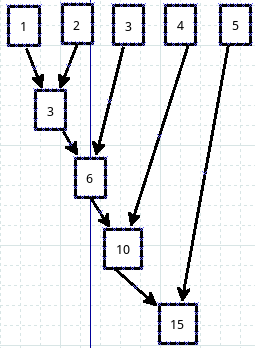# 零基础学Python:函数(4)

## 递归

``````#!/usr/bin/env python
# coding=utf-8

def fib(n):
"""
This is Fibonacci by Recursion.
"""
if n==0:
return 0
elif n==1:
return 1
else:
return fib(n-1) + fib(n-2)

if __name__ == "__main__":
f = fib(10)
print f
``````

``````\$ python 20401.py
55
``````

`fib(n-1) + fib(n-2)`就是又调用了这个函数自己，实现递归。为了明确递归的过程，下面走一个计算过程（考虑到次数不能太多，就让n=3）

1. n=3,fib(3)，自然要走`return fib(3-1) + fib(3-2)`分支
2. 先看fib(3-1),即fib(2)，也要走else分支，于是计算`fib(2-1) + fib(2-2)`
3. fib(2-1)即fib(1)，在函数中就要走elif分支，返回1，即fib(2-1)=1。同理，容易得到fib(2-2)=0。将这两个值返回到上面一步。得到`fib(3-1)=1+0=1`
4. 再计算fib(3-2),就简单了一些，返回的值是1，即fib(3-2)=1
5. 最后计算第一步中的结果：`fib(3-1) + fib(3-2) = 1 + 1 = 2`，将计算结果2作为返回值

``````#!/usr/bin/env python
# coding=utf-8

"""
the better Fibonacci
"""
meno = {0:0, 1:1}    #初始化

def fib(n):
if not n in meno:    #如果不在初始化范围内
meno[n] = fib(n-1) + fib(n-2)
return meno[n]

if __name__ == "__main__":
f = fib(10)
print f

#运行结果
\$ python 20402.py
55
``````

## 几个特殊函数

filter、map、reduce、lambda、yield

（注：本节不对yield进行介绍，请阅读《生成器》

## lambda

lambda函数，是一个只用一行就能解决问题的函数，听着是多么诱人呀。看下面的例子：

``````>>> def add(x):     #定义一个函数，将输入的变量增加3,然后返回增加之后的值
...     x += 3
...     return x
...
>>> numbers = range(10)
>>> numbers
[0, 1, 2, 3, 4, 5, 6, 7, 8, 9]  #有这样一个list，想让每个数字增加3,然后输出到一个新的list中

>>> new_numbers = []
>>> for i in numbers:
...
>>> new_numbers
[3, 4, 5, 6, 7, 8, 9, 10, 11, 12]
``````

``````>>> new_numbers = [ i+3 for i in numbers ]
>>> new_numbers
[3, 4, 5, 6, 7, 8, 9, 10, 11, 12]
``````

``````>>> lam = lambda x:x+3
>>> n2 = []
>>> for i in numbers:
...     n2.append(lam(i))
...
>>> n2
[3, 4, 5, 6, 7, 8, 9, 10, 11, 12]
``````

``````>>> g = lambda x,y:x+y  #x+y,并返回结果
>>> g(3,4)
7
>>> (lambda x:x**2)(4)  #返回4的平方
16
``````

• 在lambda后面直接跟变量
• 变量后面是冒号
• 冒号后面是表达式，表达式计算结果就是本函数的返回值

``````lambda arg1, arg2, ...argN : expression using arguments
``````

``````>>> lamb = [ lambda x:x,lambda x:x**2,lambda x:x**3,lambda x:x**4 ]
>>> for i in lamb:
...     print i(3),
...
3 9 27 81
``````

lambda做为一个单行的函数，在编程实践中，可以选择使用。

## map

``````>>> numbers
[0, 1, 2, 3, 4, 5, 6, 7, 8, 9]      #把列表中每一项都加3

[3, 4, 5, 6, 7, 8, 9, 10, 11, 12]

>>> map(lambda x: x+3,numbers)      #用lambda当然可以啦
[3, 4, 5, 6, 7, 8, 9, 10, 11, 12]
``````

map()是python的一个内置函数，它的基本样式是：

`map(func,seq)`

func是一个函数，seq是一个序列对象。在执行的时候，序列对象中的每个元素，按照从左到右的顺序，依次被取出来，并塞入到func那个函数里面，并将func的返回值依次存到一个list中。

``````>>> items = [1,2,3,4,5]
>>> squared = []
>>> for i in items:
...     squared.append(i**2)
...
>>> squared
[1, 4, 9, 16, 25]

>>> def sqr(x): return x**2
...
>>> map(sqr,items)
[1, 4, 9, 16, 25]

>>> map(lambda x: x**2, items)
[1, 4, 9, 16, 25]

>>> [ x**2 for x in items ]     #这个我最喜欢了，一般情况下速度足够快，而且可读性强
[1, 4, 9, 16, 25]
``````

• 对iterable中的每个元素，依次应用function的方法（函数）（这本质上就是一个for循环）。
• 将所有结果返回一个list。
• 如果参数很多，则对那些参数并行执行function。

``````>>> lst1 = [1,2,3,4,5]
>>> lst2 = [6,7,8,9,0]
>>> map(lambda x,y: x+y, lst1,lst2)     #将两个列表中的对应项加起来，并返回一个结果列表
[7, 9, 11, 13, 5]
``````

``````>>> lst1 = [1,2,3,4,5]
>>> lst2 = [6,7,8,9,0]
>>> lst3 = [7,8,9,2,1]
>>> map(lambda x,y,z: x+y+z, lst1,lst2,lst3)
[14, 17, 20, 15, 6]
``````

## reduce

``````>>> reduce(lambda x,y: x+y,[1,2,3,4,5])
15
````````````>>> list1 = [1,2,3,4,5,6,7,8,9]
>>> list2 = [9,8,7,6,5,4,3,2,1]
>>> map(lambda x,y: x+y, list1,list2)
[10, 10, 10, 10, 10, 10, 10, 10, 10]
``````

``````def reduce(function, iterable, initializer=None):
it = iter(iterable)
if initializer is None:
try:
initializer = next(it)
except StopIteration:
raise TypeError('reduce() of empty sequence with no initial value')
accum_value = initializer
for x in it:
accum_value = function(accum_value, x)
return accum_value
``````

``````>>> lst = range(1,6)
>>> lst
[1, 2, 3, 4, 5]
>>> r = 0
>>> for i in range(len(lst)):
...     r += lst[i]
...
>>> r
15
``````

for普世的，reduce是简洁的。

``````>>> a
[3, 9, 8, 5, 2]
>>> b
[1, 4, 9, 2, 6]

>>> zip(a,b)        #复习一下zip，下面的方法中要用到
[(3, 1), (9, 4), (8, 9), (5, 2), (2, 6)]

>>> sum(x*y for x,y in zip(a,b))    #解析后直接求和
133

>>> new_list = [x*y for x,y in zip(a,b)]    #可以看做是上面方法的分布实施

>>> #这样解析也可以：new_tuple = (x*y for x,y in zip(a,b))
>>> new_list
[3, 36, 72, 10, 12]
>>> sum(new_list)     #或者:sum(new_tuple)
133

>>> reduce(lambda sum,(x,y): sum+x*y,zip(a,b),0)    #这个方法是在耍酷呢吗？
133

>>> from operator import add,mul            #耍酷的方法也不止一个
133

>>> reduce(lambda x,y: x+y, map(lambda x,y: x*y, a,b))  #map,reduce,lambda都齐全了，更酷吗？
133
``````

## filter

filter的中文含义是“过滤器”，在python中，它就是起到了过滤器的作用。首先看官方说明：

``````>>> numbers = range(-5,5)
>>> numbers
[-5, -4, -3, -2, -1, 0, 1, 2, 3, 4]

>>> filter(lambda x: x>0, numbers)
[1, 2, 3, 4]

>>> [x for x in numbers if x>0]     #与上面那句等效
[1, 2, 3, 4]

>>> filter(lambda c: c!='i', 'qiwsir')  #能不能对应上面文档说明那句话呢？
'qwsr'                                  #“If iterable is a string or a tuple, the result also has that type;”
``````